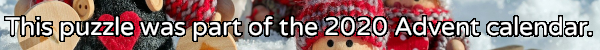mscroggs.co.uk
mscroggs.co.uksubscribe

# Puzzles

## 22 DecemberPut the digits 1 to 9 (using each digit exactly once) in the boxes so that the sums are correct. The sums should be read left to right and top to bottom ignoring the usual order of operations. For example, 4+3×2 is 14, not 10. Today's number is the largest number you can make with the digits in the red boxes.
 + + = 18 + + + ÷ - = 1/2 + + + + ÷ = 3/2 =24 =8 =13
Tags: numbers, grids

## 17 DecemberPut the digits 1 to 9 (using each digit exactly once) in the boxes so that the sums and product are correct. Today's number is the product of the numbers in the red boxes.
 + + = 16 + + + + + = 8 + + + × × = 288 =11 =14 =20
Tags: numbers, grids

## 9 DecemberPut the digits 1 to 9 (using each digit exactly once) in the boxes so that the sums are correct. The sums should be read left to right and top to bottom ignoring the usual order of operations. For example, 4+3×2 is 14, not 10. Today's number is the product of the numbers in the red boxes.
 + × = 54 × + ÷ - ÷ = 1 ÷ - × + - = 6 =18 =6 =18
Tags: numbers, grids

## 3 DecemberPut the digits 1 to 9 (using each digit exactly once) in the boxes so that the sums are correct. The sums should be read left to right and top to bottom ignoring the usual order of operations. For example, 4+3×2 is 14, not 10. Today's number is the largest number you can make with the digits in the red boxes.
 ÷ - = 3 + + ÷ ÷ × = 1 × - + - × = 20 =91 =6 =8
Tags: numbers, grids

## 23 DecemberArrange the digits 1-9 in a 3×3 square so the 3-digits numbers formed in the rows and columns are the types of numbers given at the ends of the rows and columns. The number in the first column is today's number.
 a multiple of 4 a cube a multiple of 3 today's number a cube an odd number
Tags: numbers, grids

## 21 DecemberPut the digits 1 to 9 (using each digit exactly once) in the boxes so that the sums are correct. The sums should be read left to right and top to bottom ignoring the usual order of operations. For example, 4+3×2 is 14, not 10. Today's number is the smallest number you can make with the digits in the red boxes.
 + - = 7 ÷ - ÷ + ÷ = 8 × × × + - = 7 =12 =5 =28
Tags: numbers, grids

## 16 DecemberArrange the digits 1-9 in a 3×3 square so that: the median number in the first row is 6; the median number in the second row is 3; the mean of the numbers in the third row is 4; the mean of the numbers in the second column is 7; the range of the numbers in the third column is 2, The 3-digit number in the first column is today's number.
 median 6 median 3 mean 4 today's number mean 7 range 2

## 11 DecemberPut the digits 1 to 9 (using each digit exactly once) in the boxes so that the sums are correct. The sums should be read left to right and top to bottom ignoring the usual order of operations. For example, 4+3×2 is 14, not 10. Today's number is the product of the red digits.
 + ÷ = 2 + ÷ ÷ ÷ ÷ = 3 ÷ - ÷ ÷ ÷ = 1 =2 =1 =1
Tags: grids, numbers

## Archive

Show me a random puzzle
▼ show ▼